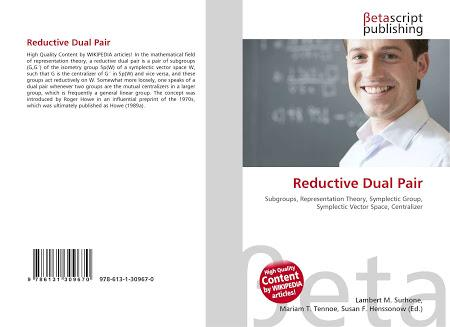What Do You Know About Reductive Dual Pairs?

10 QuestionsSettingsThis is part of the representation theory in mathematics. A reductive dual pair is termed as a pair of two subgroups under the isometry group. After the introduction of the theorem, it has been effectively used for mathematical expressions and calculations. In this quiz, you will learn more about the basic concepts of this theorem.

• 1.
Who introduced the theorem?
• A.

Roger Howe

• B.

Matt Ashley

• C.

Thomas Howe

• D.

Clifford Griffin

• 2.
When was it introduced?
• A.

1960s

• B.

1970s

• C.

1980s

• D.

1990s

• 3.
When was it published?
• A.

1989

• B.

1969

• C.

1979

• D.

1999

• 4.
What does Sp(W) represent?
• A.

Glitzla group

• B.

Spectial group

• C.

Sub-group

• D.

Isometry group

• 5.
What does X represent?
• A.

N-dimensional vector space

• B.

Dual

• C.

Direction

• D.

Space

• 6.
What does Y represent?
• A.

N-dimensional vector space

• B.

Dual

• C.

Direction

• D.

Space

• 7.
What does W represent?
• A.

Differences between X and Y

• B.

Sum of X and Y

• C.

Multiplication of X and Y

• D.

X/Y

• 8.
What does G represent in G′ of Sp(W)?
• A.

Decentralizer

• B.

Centralizer

• C.

Group

• D.

Free space

• 9.
What does G denote?
• A.

Two-element group

• B.

Full symplectic group

• C.

Subgroup

• D.

Complete group

• 10.
What does G′ represent?
• A.

Two-element group

• B.

Full symplectic group

• C.

Subgroup

• D.

Complete group

Related Topics